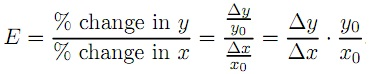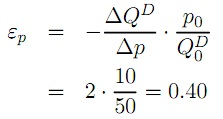#### Elasticity

Elasticity:

Elasticity is always a ratio between two percentage changes. For a general expression, y = f(x), the elasticity of y with respect to x is:Note that the term ?y/?x shows the marginal effect, or slope of the function in the x-direction, and (y0, x0) is the starting point. For a linear function the slope is always constant, but the elasticity is not constant because it varies with the starting point.

Example:

As an example, consider the following demand function for beer:

QD = 100 − 2. p + 0.05. ps − 0.25 . pc − 0.00125 . Y

QD is here expressed in a volume measure (liters or bottles or cans etc) per month and prices and income in money (kr, €, \$, £ etc). The substitute good maybe wine and the complement good sausage. If p = 10kr/bottle, ps = 50kr/bottle, pc = 30kr/kilo and Y = 20000kr/month. Inserting these numbers:

QD = 100 – 2 x 10 + 0.05 x 50 − 0.25 x 30 − 0.00125 x 20000 = 50,

i.e., this household, or consumer, demands 50liters of beer per month. Now, the demand curve is a graph which shows the relationship between the own price and quantity demanded, so if we aggregate all the numbers above except for the price we get:

Q = (100 + 0.05 x 50 − 0.25x 30 − 0.00125 x 20000) − 2 x p
Q = 70 − 2. p

QD = 70 − 2. p

Given that the assumed value of the all other variables except the price. The marginal effect is −2, and if we start at the equilibrium we calculated initially,

i.e. p0 = 10, Q0 = 50, the price elasticity is,This means that if the price changes by one percent, the quantity demanded changes by 0.4 percent. If the own price elasticity is less than 1 (after changing sign, since the marginal effect is always negative), we say that demand is inelastic.

Latest technology based Economics Online Tutoring Assistance

Tutors, at the www.tutorsglobe.com, take pledge to provide full satisfaction and assurance in Intermediate Microeconomics homework help via online tutoring. Students are getting 100% satisfaction by online tutors across the globe. Here you can get homework help for Intermediate Microeconomics, project ideas and tutorials. We provide email based Intermediate Microeconomics homework help. You can join us to ask queries 24x7 with live, experienced and qualified online tutors specialized in Intermediate Microeconomics. Through Online Tutoring, you would be able to complete your homework or assignments at your home. Tutors at the TutorsGlobe are committed to provide the best quality online tutoring assistance for Economics homework help and assignment help services. They use their experience, as they have solved thousands of the Economics assignments, which may help you to solve your complex issues of Intermediate Microeconomics. TutorsGlobe assure for the best quality compliance to your homework. Compromise with quality is not in our dictionary. If we feel that we are not able to provide the homework help as per the deadline or given instruction by the student, we refund the money of the student without any delay.